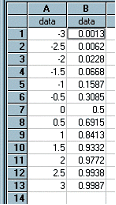﻿ Normal(column) / NormP(column)

# Normal(column) / NormP(column)

Top  Previous  Next
 These two functions are equivalent.   The data in column is interpreted as z-scores (standard deviations) and the Normal function calculates the probability that a score will be less than or equal to the z-score in column.  This function is equivalent to the P function on many scientific calculators or the normal distribution data in a tables book.   The data in column B of the example has been generated using Normal(A)